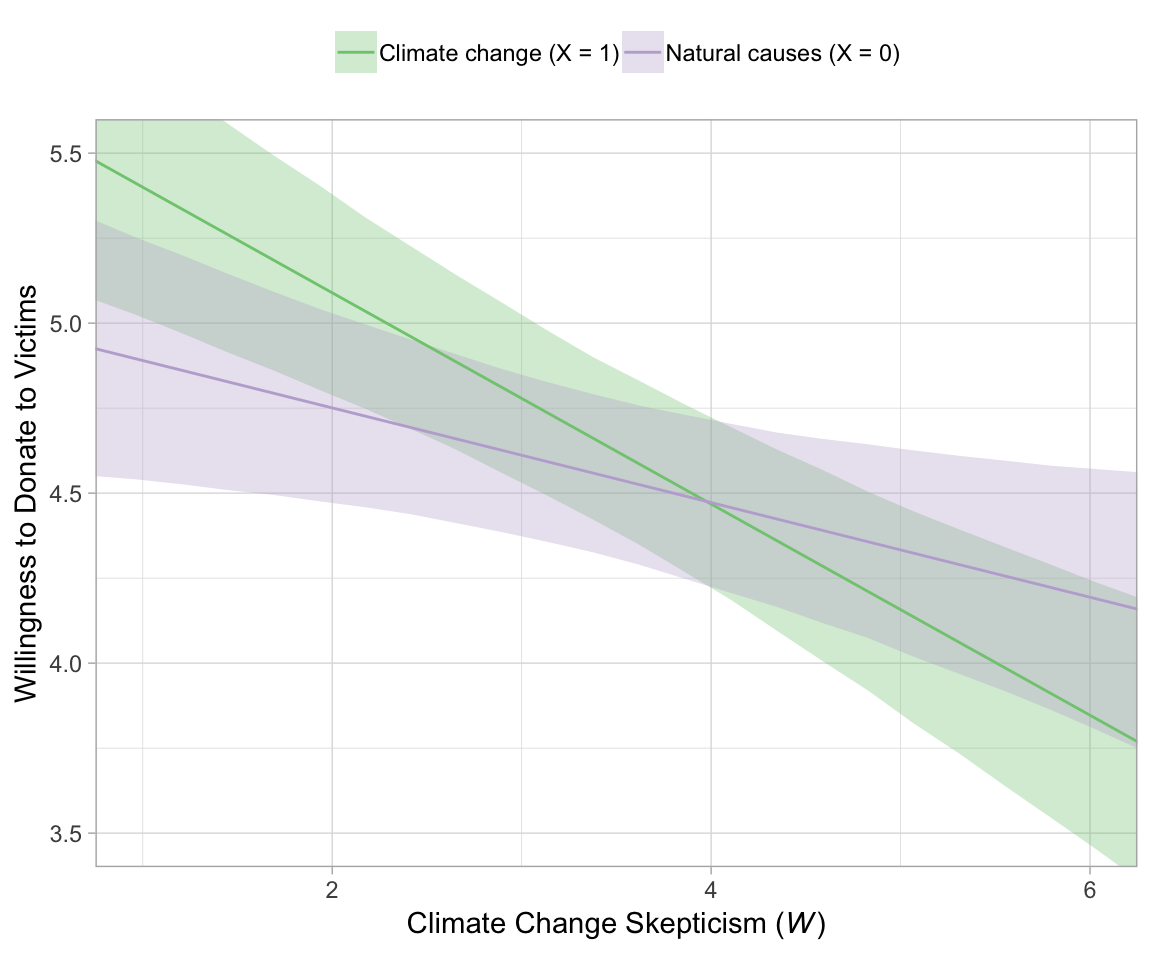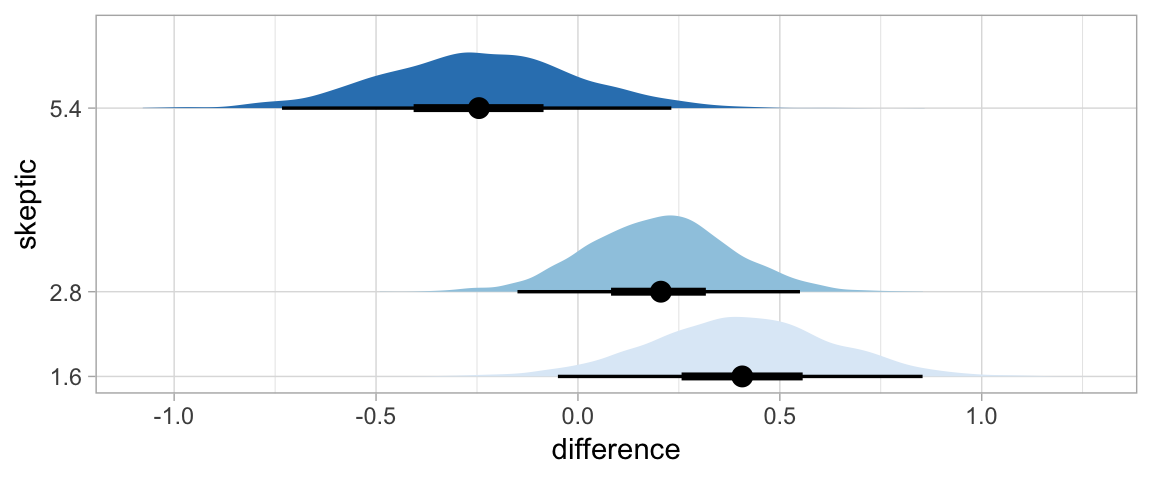## 12.1 Revisiting the disaster framing study

Here we load a couple necessary packages, load the data, and take a glimpse().

library(tidyverse)

disaster <- read_csv("data/disaster/disaster.csv")

glimpse(disaster)
## Observations: 211
## Variables: 5
## $id <int> 1, 2, 3, 4, 5, 6, 7, 8, 9, 10, 11, 12, 13, 14, 15, 16, 17, 18, 19, 20, 21, 22, 23, 24, 25... ##$ frame   <int> 1, 1, 1, 1, 1, 0, 0, 1, 0, 0, 1, 1, 0, 0, 1, 1, 1, 1, 0, 0, 1, 0, 1, 0, 1, 1, 0, 0, 0, 1,...
## $donate <dbl> 5.6, 4.2, 4.2, 4.6, 3.0, 5.0, 4.8, 6.0, 4.2, 4.4, 5.8, 6.2, 6.0, 4.2, 4.4, 5.8, 5.4, 3.4,... ##$ justify <dbl> 2.95, 2.85, 3.00, 3.30, 5.00, 3.20, 2.90, 1.40, 3.25, 3.55, 1.55, 1.60, 1.65, 2.65, 3.15,...
## $skeptic <dbl> 1.8, 5.2, 3.2, 1.0, 7.6, 4.2, 4.2, 1.2, 1.8, 8.8, 1.0, 5.4, 2.2, 3.6, 7.8, 1.6, 1.0, 6.4,... Load brms. library(brms) model1 is the simple moderation model. model1 <- brm(data = disaster, family = gaussian, donate ~ 1 + frame + skeptic + frame:skeptic, chains = 4, cores = 4) Our model1 summary matches nicely with the text. print(model1, digits = 3) ## Family: gaussian ## Links: mu = identity; sigma = identity ## Formula: donate ~ 1 + frame + skeptic + frame:skeptic ## Data: disaster (Number of observations: 211) ## Samples: 4 chains, each with iter = 2000; warmup = 1000; thin = 1; ## total post-warmup samples = 4000 ## ## Population-Level Effects: ## Estimate Est.Error l-95% CI u-95% CI Eff.Sample Rhat ## Intercept 5.029 0.228 4.584 5.479 2070 1.002 ## frame 0.681 0.335 0.014 1.333 1969 1.001 ## skeptic -0.139 0.058 -0.255 -0.025 1949 1.003 ## frame:skeptic -0.171 0.085 -0.341 0.003 1816 1.002 ## ## Family Specific Parameters: ## Estimate Est.Error l-95% CI u-95% CI Eff.Sample Rhat ## sigma 1.240 0.062 1.124 1.366 2855 1.001 ## ## Samples were drawn using sampling(NUTS). For each parameter, Eff.Sample ## is a crude measure of effective sample size, and Rhat is the potential ## scale reduction factor on split chains (at convergence, Rhat = 1). For the figures in this chapter, we’ll take theme cues from Matthew Kay’s tidybayes package. Otherwise, our Figure 12.2 is business as usual at this point. theme_set(theme_light()) nd <- tibble(frame = rep(0:1, each = 30), skeptic = rep(seq(from = 0, to = 7, length.out = 30), times = 2)) fitted(model1, newdata = nd) %>% as_tibble() %>% bind_cols(nd) %>% mutate(frame = ifelse(frame == 0, str_c("Natural causes (X = ", frame, ")"), str_c("Climate change (X = ", frame, ")"))) %>% ggplot(aes(x = skeptic, y = Estimate)) + geom_ribbon(aes(ymin = Q2.5, ymax = Q97.5, fill = frame), alpha = 1/3) + geom_line(aes(color = frame)) + scale_fill_brewer(type = "qual") + scale_color_brewer(type = "qual") + coord_cartesian(xlim = 1:6, ylim = c(3.5, 5.5)) + labs(x = expression(paste("Climate Change Skepticism (", italic(W), ")")), y = "Willingness to Donate to Victims") + theme(legend.position = "top", legend.direction = "horizontal", legend.title = element_blank())In Hayes’s Figure 12.2, he emphasized the differences at the three levels of skeptic. If you want the full difference score distributions in a pick-a-point-approach sort of way, you might plot the densities with tidybayes::geom_halfeyeh(), which places coefficient plots at the base of the densities. In this case, we show the posterior medians with the dots, the 50% intervals with the thick horizontal lines, and the 95% intervals with the thinner horizontal lines. library(tidybayes) nd <- tibble(frame = rep(0:1, times = 3), skeptic = rep(quantile(disaster$skeptic, probs = c(.16, .5, .86)),
times = 2))

fitted(model1, summary = F,
newdata = nd) %>%
as_tibble() %>%
gather() %>%
mutate(frame = rep(rep(0:1, times = 3),
each = 4000),
skeptic = rep(rep(quantile(disaster$skeptic, probs = c(.16, .5, .86)), times = 2), each = 4000), iter = rep(1:4000, times = 6)) %>% select(-key) %>% spread(key = frame, value = value) %>% mutate(difference = 1 - 0) %>% ggplot(aes(x = difference, y = skeptic, fill = skeptic %>% as.character())) + geom_halfeyeh(point_interval = median_qi, .prob = c(0.95, 0.5)) + scale_fill_brewer() + scale_y_continuous(breaks = quantile(disaster$skeptic, probs = c(.16, .5, .86)),
labels = quantile(disaster\$skeptic, probs = c(.16, .5, .86)) %>% round(2)) +
theme(legend.position = "none",
panel.grid.minor.y = element_blank())Here’s our simple mediation model, model2, using the multivariate syntax right in the brm() function.

model2 <-
brm(data = disaster, family = gaussian,
bf(justify ~ 1 + frame) +
bf(donate ~ 1 + frame + justify) +
set_rescor(FALSE),
chains = 4, cores = 4)
print(model2, digits = 3)
##  Family: MV(gaussian, gaussian)
##   Links: mu = identity; sigma = identity
##          mu = identity; sigma = identity
## Formula: justify ~ 1 + frame
##          donate ~ 1 + frame + justify
##    Data: disaster (Number of observations: 211)
## Samples: 4 chains, each with iter = 2000; warmup = 1000; thin = 1;
##          total post-warmup samples = 4000
##
## Population-Level Effects:
##                   Estimate Est.Error l-95% CI u-95% CI Eff.Sample  Rhat
## justify_Intercept    2.801     0.091    2.619    2.981       4000 1.000
## donate_Intercept     7.235     0.233    6.776    7.688       4000 0.999
## justify_frame        0.134     0.127   -0.116    0.379       4000 1.000
## donate_frame         0.211     0.135   -0.060    0.480       4000 1.000
## donate_justify      -0.954     0.076   -1.102   -0.805       4000 0.999
##
## Family Specific Parameters:
##               Estimate Est.Error l-95% CI u-95% CI Eff.Sample  Rhat
## sigma_justify    0.935     0.045    0.850    1.026       4000 1.000
## sigma_donate     0.987     0.049    0.898    1.090       4000 0.999
##
## Samples were drawn using sampling(NUTS). For each parameter, Eff.Sample
## is a crude measure of effective sample size, and Rhat is the potential
## scale reduction factor on split chains (at convergence, Rhat = 1).

Consider the Bayesian $$R^2$$ summaries.

bayes_R2(model2) %>% round(digits = 3)
##            Estimate Est.Error  Q2.5 Q97.5
## R2_justify    0.010     0.011 0.000 0.040
## R2_donate     0.449     0.039 0.367 0.519

If you want the indirect effect with its intervals, you use posterior_samples() and data wrangle, as usual.

posterior_samples(model2) %>%
mutate(ab = b_justify_frame*b_donate_justify) %>%
summarize(mean = mean(ab),
ll = quantile(ab, probs = .025),
ul = quantile(ab, probs = .975)) %>%
mutate_if(is.double, round, digits = 3)
##     mean     ll   ul
## 1 -0.128 -0.364 0.11

We might also streamline our code a touch using tidybayes::mean_qi() in place of tidyverse::summarize().

posterior_samples(model2) %>%
mutate(ab = b_justify_frame*b_donate_justify) %>%
mean_qi(ab, .prob = .95) %>%
mutate_if(is.double, round, digits = 3)
## # A tibble: 1 x 4
##       ab conf.low conf.high .prob
##    <dbl>    <dbl>     <dbl> <dbl>
## 1 -0.128   -0.364      0.11  0.95

Note that the last column explicates what interval level we used.